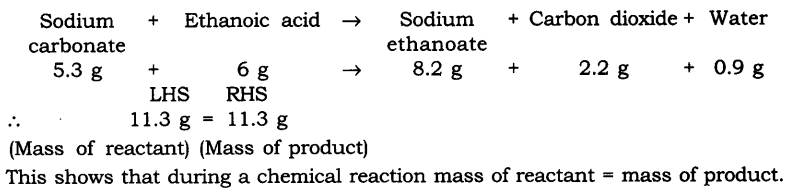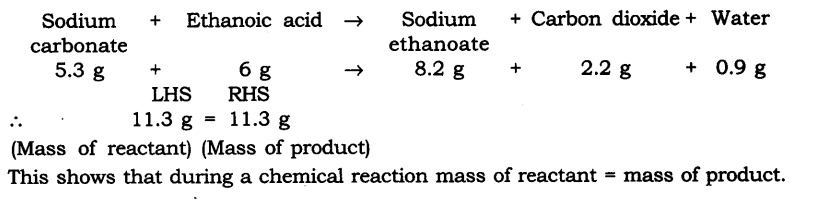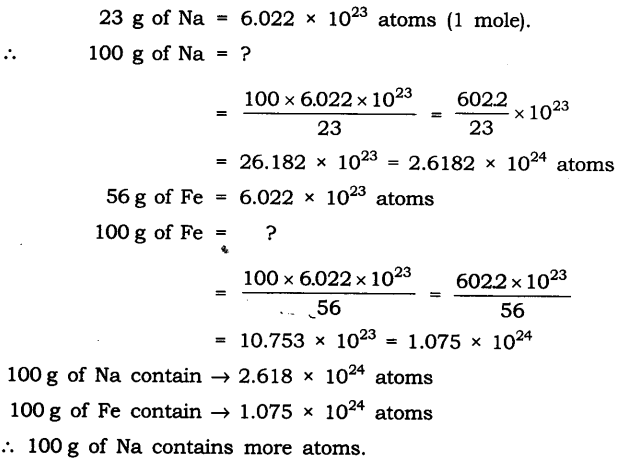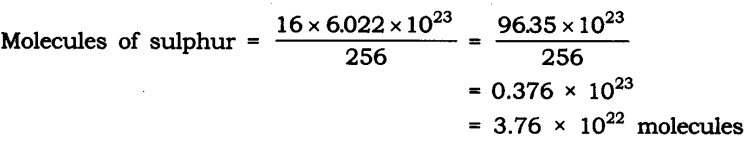# NCERT Solutions For Class 9 Science Chapter 3 Atoms and Molecules

NCERT Solutions For Class 9 Science Chapter 3 Atoms and Molecules

IN-TEXT QUESTIONS SOLVED

NCERT Textbook for Class 9 Science – Page 32
Question 1. In a reaction 5.3 g of sodium carbonate reacted with 6 g of ethanoic acid. The products were 2.2 g of carbon dioxide, 0.9 g water and 8.2 g of sodium ethanoate. Show that these observations are in agreement with the law of conservation of mass carbonate.CBSE Class 9 Science Chapter 3 IN-TEXT QUESTIONS SOLVED

Class 9 Science NCERT Textbook Page 32
Question 1. In a reaction 5.3 g of sodium carbonate reacted with 6 g of ethanoic acid. The products were 2.2 g of carbon dioxide, 0.9 g water and 8.2 g of sodium etkanoate. Show that these observations are in agreement with the law of conservation of mass carbonate.Question 2. Hydrogen and oxygen combine in the ratio of 1 : 8 by mass to form water. What mass of oxygen gas would be required to react completely with 3 g of hydrogen gas?
Answer: Ratio of H : O by mass in water is:
Hydrogen : Oxygen —> H2O
∴ 1 : 8 = 3 : x
x = 8 x 3
x = 24 g
∴ 24 g of oxygen gas would be required to react completely with 3 g of hydrogen gas.

Question 3. Which postulate of Dalton’s atomic theory is the result of the law of conservation of mass?
Answer: The postulate of Dalton’s atomic theory that is the result of the law of conservation of mass is—the relative number and kinds of atoms are constant in a given compound. Atoms cannot be created nor destroyed in a chemical reaction.

Question 4. Which postulate of Dalton’s atomic theory can explain the law of definite proportions?
Answer: The relative number and kinds of atoms are constant in a given compound.

Class 9 Science NCERT Textbook Page 35
Question 1. Define the atomic mass unit.
Answer: One atomic mass unit is equal to exactly one-twelfth (1/12th) the mass of one atom of carbon-12. The relative atomic masses of all elements have been found with respect to an atom of carbon-12.

Question 2. Why is it not possible to see an atom with naked eyes?
Answer: Atom is too small to be seen with naked eyes. It is measured in nanometres.
1 m = 109 nm

NCERT Textbook Questions – Page 39
Question 1. Write down the formulae of
(i) Sodium oxide
(ii) Aluminium chloride
(iii) Sodium sulphide
(iv) Magnesium hydroxideQuestion 2. What is meant by the term chemical formula?
Answer: The chemical formula of the compound is a symbolic representation of its composition, e.g., chemical formula of sodium chloride is NaCl.

Question 3. How many atoms are present in a
(i) H2S molecule and
(ii) P043- ion?
Answer: (i) H2S —> 3 atoms are present
(ii) P043- —> 5 atoms are present

NCERT Textbook Questions – Page 40
Question 1. Calculate the molecular masses of H2, O2, Cl2, C02, CH4, C2H2,NH3, CH3OH.Question 2.Calculate the formula unit masses of ZnO, Na2O, K2C03, given atomic masses of Zn = 65 u, Na = 23 u, K = 39 u, C = 12 u, and O = 16 u.
Answer: The formula unit mass of
(i) ZnO = 65 u + 16 u = 81 u
(ii) Na2O = (23 u x 2) + 16 u = 46 u + 16 u = 62 u
(iii) K2C03 = (39 u x 2) + 12 u + 16 u x 3
= 78 u + 12 u + 48 u = 138 u

Class 9 Science NCERT Textbook Page 42
Question 1. If one mole of carbon atoms weigh 12 grams, what is the mass (in grams) of 1 atom of carbon?Question 2. Which has more number of atoms, 100 grams of sodium or 100 grams of iron (given atomic mass of Na = 23 u, Fe = 56 u)?Questions From NCERT Textbook for Class 9 Science

Question 1. A 0.24 g sample of compound of oxygen and boron was found by analysis to contain 0.096 g of boron and 0.144 g of oxygen. Calculate the percentage composition of the compound by weight.
Answer: Boron and oxygen compound —> Boron + Oxygen
0.24 g —> 0.096 g + 0.144 gQuestion 2. When 3.0 g of carbon is burnt in 8.00 g oxygen, 11.00 g of carbon dioxide is produced. What mass of carbon dioxide will be formed when 3.00 g of carbon is burnt in 50.00 g of oxygen? Which law of chemical combination will govern your answer?
Answer: The reaction of burning of carbon in oxygen may be written as:It shows that 12 g of carbon bums in 32 g oxygen to form 44 g of carbon dioxide. Therefore 3 g of carbon reacts with 8 g of oxygen to form 11 g of carbon dioxide. It is given that 3.0 g of carbon is burnt with 8 g of oxygen to produce 11.0 g of CO2. Consequently 11.0 g of carbon dioxide will be formed when 3.0 g of C is burnt in 50 g of oxygen consuming 8 g of oxygen, leaving behind 50 – 8 = 42 g of O2. The answer governs the law of constant proportion.

Question 3. What are poly atomic ions? Give examples.
Answer: The ions which contain more than one atoms (same kind or may be of different kind) and behave as a single unit are called polyatomic ions e.g., OH, SO42-, CO32-.

Question 4. Write the chemical formulae of the following:
(a) Magnesium chloride
(b) Calcium oxide
(c) Copper nitrate
(d) Aluminium chloride
(e) Calcium carbonate.
Symbol —> Mg Cl
Change —> +2 -1
Formula —> MgCl2
(b) Calcium oxide
Symbol —> Ca O
Charge —> +2 -2
Formula —> CaO
(c) Copper nitrate
Symbol —> Cu NO
Change +2 -1
Formula -4 CU(N03)2
(d) Aluminium chloride
Symbol —> Al Cl
Change —> +3 -1
Formula —> AlCl3
(d) Calcium carbonate
Symbol —> Ca CO3
Change —> +2 -2
Formula —> CaC03

Question 5. Give the names of the elements present in the following compounds:
(a) Quick lime
(b) Hydrogen bromide
(c) Baking powder
(d) Potassium sulphate.
Answer: (a) Quick lime —> Calcium oxide
Elements —> Calcium and oxygen
(b) Hydrogen bromide
Elements —> Hydrogen and bromine
(c) Baking powder —> Sodium hydrogen carbonate
Elements —> Sodium, hydrogen, carbon and oxygen
(d) Potassium sulphate
Elements —> Potassium, sulphur and oxygen

Question 6. Calculate the molar mass of the following substances.
(a) Ethyne, C2H2
(b) Sulphur molecule, S8
(c) Phosphorus molecule, P4 (Atomic mass of phosphorus = 31)
(d) Hydrochloric acid, HCl
(e) Nitric acid, HNO3
Answer: The molar mass of the following: [Unit is ‘g’]
(a) Ethyne, C2H2 = 2 x 12 + 2 x 1 = 24 + 2 = 26 g
(b) Sulphur molecule, S8 = 8 x 32 = 256 g
(c) Phosphorus molecule, P4=4 x 31 = i24g
(d) Hydrochloric acid, HCl = 1 x 1 + 1 x 35.5 = 1 + 35.5 = 36.5 g
(e) Nitric acid, HN03 = 1 x 1 + 1 x 14 + 3 x 16 = 1 + 14 + 48 = 63 g

Question 7. What is the mass of
(a) 1 mole of nitrogen atoms?
(b) 4 moles of aluminium atoms (Atomic mass of aluminium = 27)?
(c) 10 moles of sodium sulphite (Na2S03)?
Answer: (a) Mass of 1 mole of nitrogen atoms = 14 g
(b) 4 moles of aluminium atoms
Mass of 1 mole of aluminium atoms = 27 g
∴ Mass of 4 moles of aluminium atoms = 27 x 4 = 108 g
(c) 10 moles of sodium sulphite (Na2SO3)
Mass of 1 mole of Na2SO3 = 2 x 23 + 32 + 3 x 16 = 46 + 32 + 48 = 126 g
∴ Mass of 10 moles of Na2SO3 = 126 x 10 = 1260 g

Question 8. Convert into mole.
(a) 12 g of oxygen gas
(b) 20 g of water
(c) 22 g of Carbon dioxide.
Answer: (a) Given mass of oxygen gas = 12 g
Molar mass of oxygen gas (O2) = 32 g
Mole of oxygen gas 12/32 = 0.375 mole
(b) Given mass of water = 20 g
Molar mass of water (H2O) = (2 x 1) + 16 = 18 g
Mole of water = 20/18 = 1.12 mole
(c) Given mass of Carbon dioxide = 22 g
Molar mass of carbon dioxide (CO2) = (1 x 12) + (2 x 16)
= 12 + 32 = 44 g
∴ Mole of carbon dioxide = 22/44 = 0.5 mole

Question 9. What is the mass of:
(a) 0.2 mole of oxygen atoms?
(b) 0.5 mole of water molecules?
Answer: (a) Mole of Oxygen atoms = 0.2 mole
Molar mass of oxygen atoms = 16 g
Mass of oxygen atoms = 16 x 0.2 = 3.2 g
(b) Mole of water molecule = 0.5 mole
Molar mass of water molecules = 2 x 1 + 16= 18 g .
Mass of H2O = 18 x 0.5 = 9 g

Question 10. Calculate the number of molecules of sulphur (S8) present in 16 g of solid sulphur.
Answer: Molar mass of S8 sulphur = 256 g = 6.022 x 1023 molecule
Given mass of sulphur = 16 gQuestion 11. Calculate the number of aluminium ions present in 0.051 g of aluminium oxide. (Hint: The mass of an ion is the same as that of an atom of the same element. Atomic mass of Al = 27 u)
Answer: Molar mass of aluminium oxide Al203
= (2 x 27) + (3 x 16)
= 54 + 48 = 102 g.## SabDekho

The Complete Educational Website